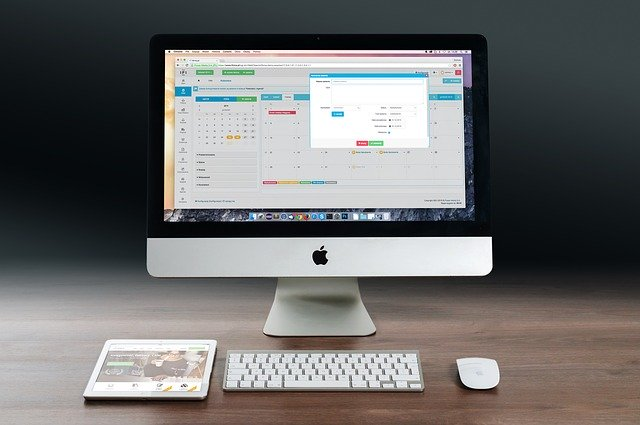# Decision tree using R Language

INTRODUCTION

The report conveys the visualization of data using the R language and it will consider the big data for visualizing the data. In addition, the report will show how to make the decision using this data set. Finally, the report will discuss the advantage and purpose of the decision tree and the implementation of this dataset using Rstudio and r language.

DISCUSSION

In order to do the data visualization, the report considered the big data of Portuguese bank data and it is mainly used to show the telemarketing calls prediction for selling the long-term deposit of bank. Here, used the data science and big data analysis concept. Main aim of the assignment is to make the decision tree based on the given dataset.

The actual definition of the decision tree is to represent the graph, which helps to illustrate each outcomes of any decision by using the branching based method. Moreover, the decision tree especially helps to provide any kind of decision and the output just looks like a tree. The several chances of outcomes and impact of each decision.

With the help of RSTUDIO and R language, the decision tree is made for showing the decision for the bank dataset (Gould, 2019). In the programming, by using R programming, at first read the dataset and then made the train dataset and test to make the decision tree. Here, different types of library or packages are used such as dplyr, rpart, rpart.plot, tree etc (Loraine et al., 2015).

in addition, inbuilt function is used along with some new created functions. The main purpose of the rpart() is to perform the tenfold cross validation using the dataset (Nijhawan et al., 2019). Raprt.plot is used to draw the actual decision tree including the text that is showing within the decision tree plot.

create_train_test & lt;- function(data, size = 0.8, train = TRUE){

n_row = nrow(data)

total_row = size * n_row

train_sample & lt; -1: total_row

if(train == TRUE){

return (data[train_sample, ])

} else {

return (data[-train_sample, ])

}

}

Above-mentioned function can be used to generate the test case and train set of data and based on these two datasets, the decision tree can be drawn.

Output

CONCLUSION

It is concluded from the above that, the report showed the use of RSTUDIO and R language in the data science and visualization of data. The Portuguese bank is selected for doing this kind of visualization.

The visualization consists of various types of representation data using graph, plot and in this case, decision tree is used to show this visualization of big data. The reports choose R language and it is appropriate to do this task. In future, this visualization of big data and R will help in other case of data science.

REFERENCES

Gould, S.J., 2019, April. Bespoke Data Visualization using R and ggplot2. In Extended Abstracts of the 2019 CHI Conference on Human Factors in Computing Systems (p. C22). ACM.

Loraine, A.E., Blakley, I.C., Jagadeesan, S., Harper, J., Miller, G. and Firon, N., 2015. Analysis and visualization of RNA-Seq expression data using RStudio, Bioconductor, and Integrated Genome Browser. In Plant Functional Genomics (pp. 481-501). Humana Press, New York, NY.

Nijhawan, V.K., Madan, M. and Dave, M., 2019. An Analytical Implementation of CART Using RStudio for Churn Prediction. In Information and Communication Technology for Competitive Strategies (pp. 109-120). Springer, Singapore.